# Comparing Ratios Unit 1 Lesson 4 Math 6

• Slides: 21Comparing Ratios Unit 1 Lesson 4 Math 6Comparing Ratios Students will be able to: Compare ratios using different methods. Make decisions by comparing ratios.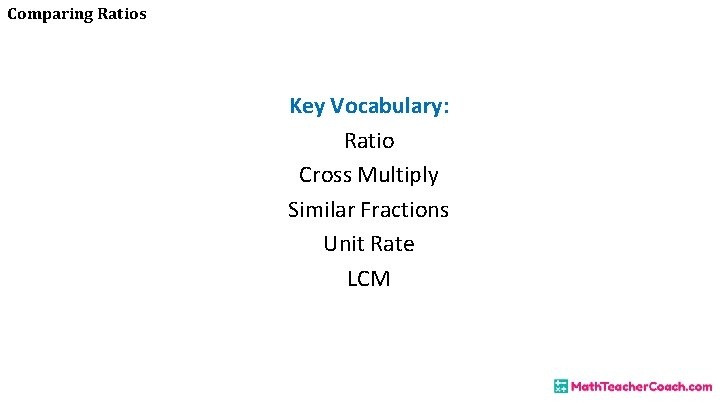Comparing Ratios Key Vocabulary: Ratio Cross Multiply Similar Fractions Unit Rate LCMComparing Ratios Comparison of Ratios A ratio is a comparison of two quantities. To compare ratios is to determine whether the two given ratios are equal or not, and if one is greater or lesser than the other. This is very helpful in making decisions in real life situations. These comparisons can be obtained using different methods.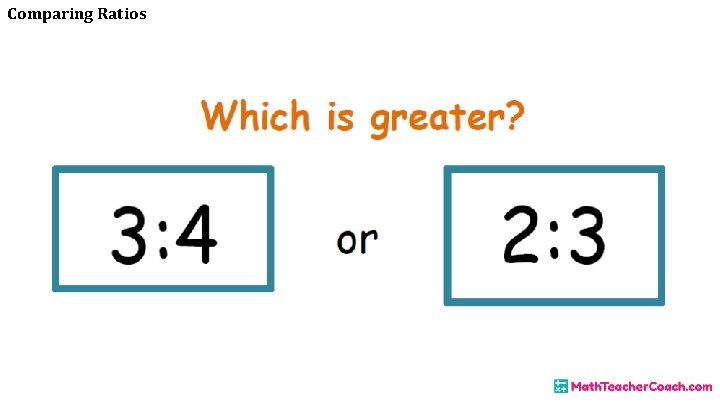Comparing RatiosComparing Ratios Method 1: COMPARING BY CROSS MULTIPLICATION Remember that ratios can be written as fractions, so one way to compare them is through the process of cross multiplying.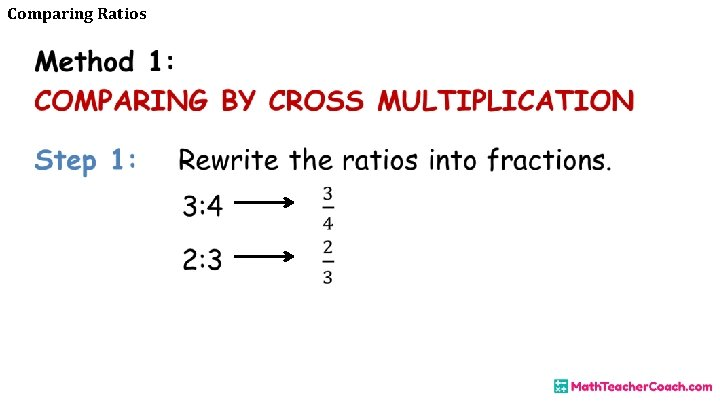Comparing Ratios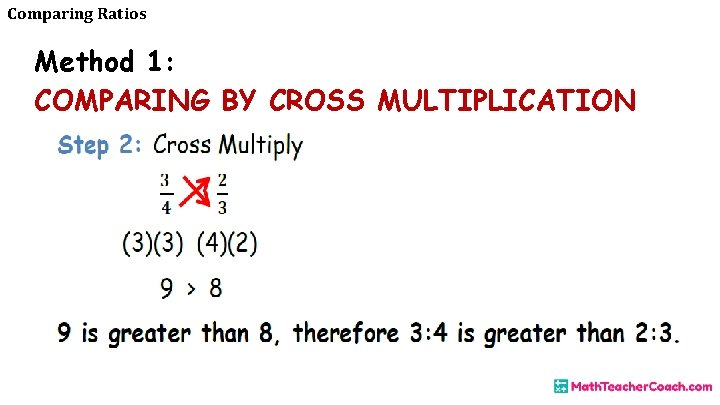Comparing Ratios Method 1: COMPARING BY CROSS MULTIPLICATION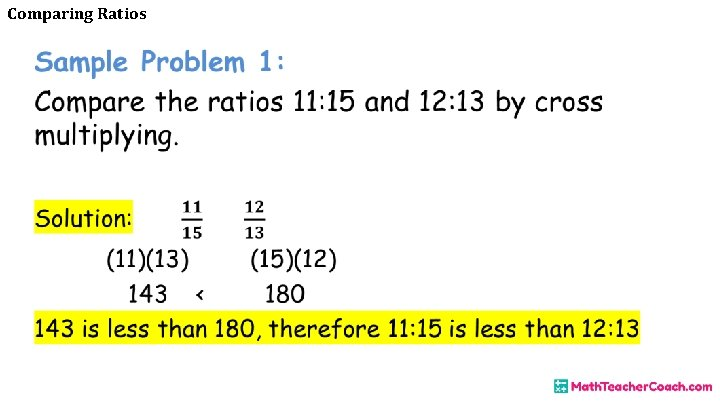Comparing RatiosComparing Ratios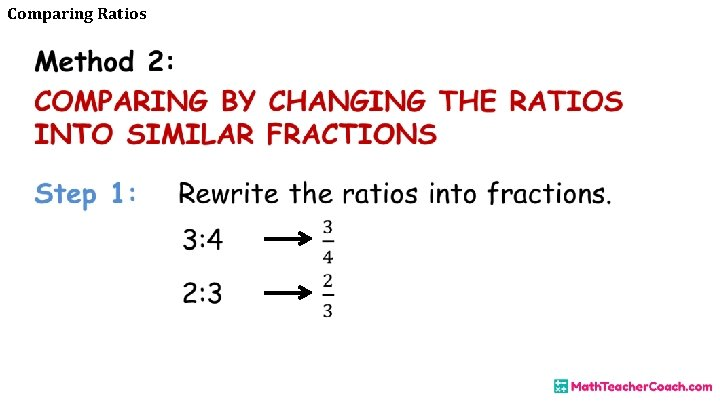Comparing RatiosComparing Ratios Method 2: COMPARING BY CHANGING THE RATIOS INTO SIMILAR FRACTIONSComparing Ratios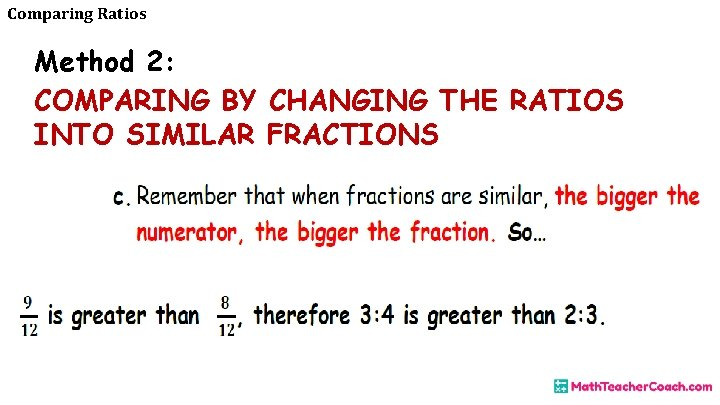Comparing Ratios Method 2: COMPARING BY CHANGING THE RATIOS INTO SIMILAR FRACTIONSComparing Ratios Sample Problem 2: Compare the ratios 4: 5 and 1: 3 by changing them into similar fractions.Comparing Ratios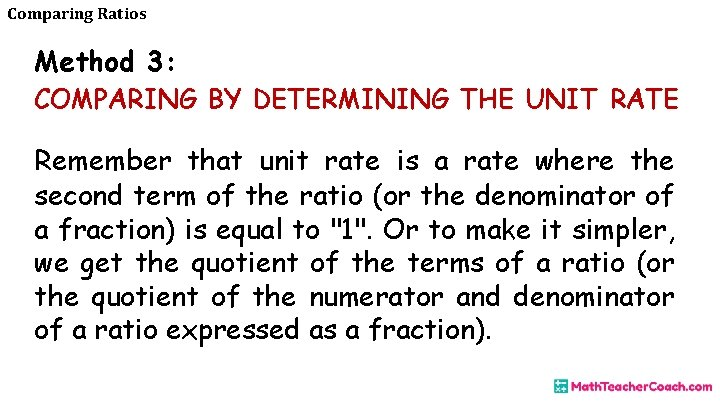Comparing Ratios Method 3: COMPARING BY DETERMINING THE UNIT RATE Remember that unit rate is a rate where the second term of the ratio (or the denominator of a fraction) is equal to "1". Or to make it simpler, we get the quotient of the terms of a ratio (or the quotient of the numerator and denominator of a ratio expressed as a fraction).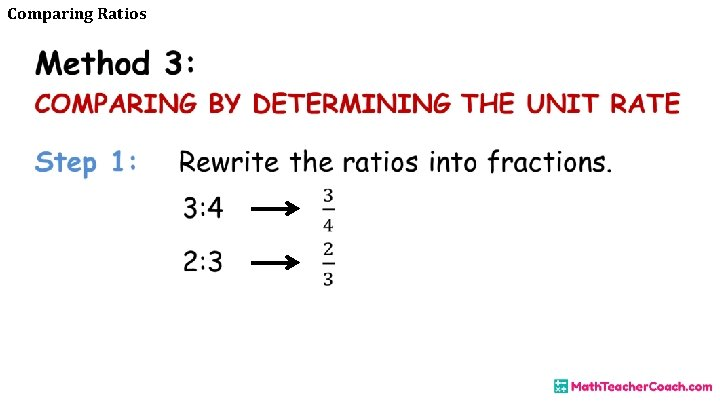Comparing Ratios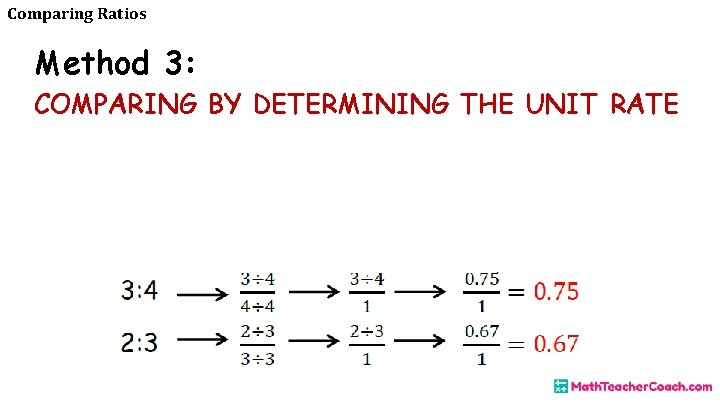Comparing Ratios Method 3: COMPARING BY DETERMINING THE UNIT RATEComparing RatiosComparing Ratios Sample Problem 3: Compare the ratios 4: 3 and 7: 5 by determining the unit rate.